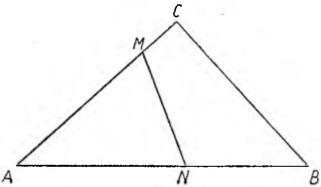# A smart division of the lotTwo brothers inherited from their father a large square in the shape of a triangle, surrounded by trees. They decided to divide it into equal parts in a straight line like this, so that the shortest possible fence could be placed on the common border.

The work of the surveyor was not easy, to which they made such a request. However, he began to remember various geometric patterns and finally found a place, in which it was necessary to draw the border. Let us follow his reasoning:

Of all the triangles with a given base and a given angle at the vertex, an isosceles triangle will have the greatest area, because the geometric location of the vertices of such triangles will be the arc containing the given angle, and the highest point of the arc is at its center. So the opposite can be said, that of all triangles with a given area and a given angle at the vertex, an isosceles triangle has the smallest base. In turn of all isosceles triangles with a given area, this area will have the smallest base, whose angle at the vertex will be the smallest.

We also know from elementary geometry, that the areas of two triangles having one common angle are so to each other, like the products of the sides making up that common angle.On the basis of the above premises, ABC belonged in a triangular plot (see the drawing) from vertex A., whose angle is the smallest, set aside along both sides the sections AM = AN equal to the proportional average between one of the sides AB or AC and the half of the other side. The MN line will be the line of the boundary you are looking for, because
Δ AMN : Δ ABC = (AM • AN) : (AC • AB),

it means

Δ AMN : Δ ABC = ½,

so the triangle AMN is half of the triangle ABC.